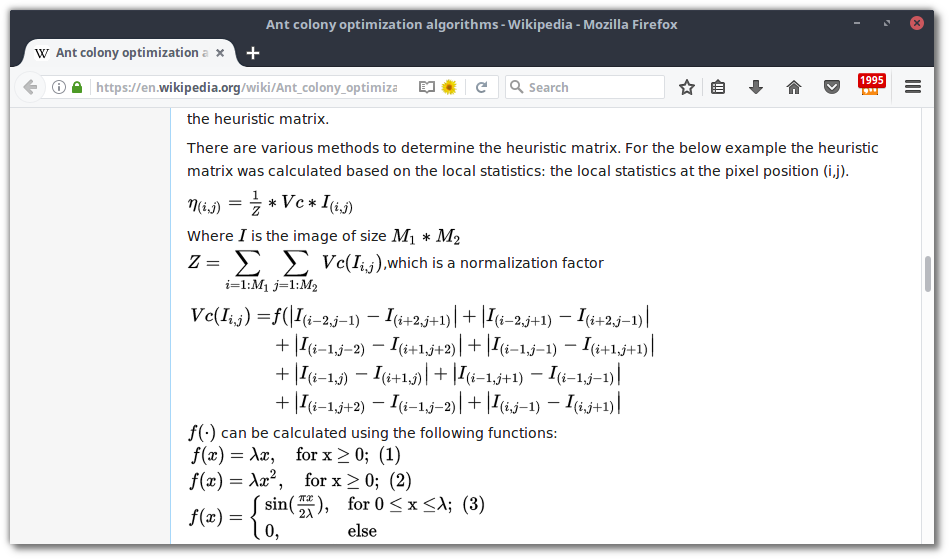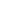| lesson material | material summary | questions and answers | definitions | types and examples | other information | materi pelajaran | ringkasan materi | pertanyaan dan jawaban | definisi | jenis-jenis dan contoh-contoh | informasi lainnya |

# LaTeX Code for Writing Mathematical Formulas in HTMLHTML itself has a tag for writing mathematical formulas. But it's long enough to use it.
Therefore, so that it doesn't bother, we can use the LaTex code only with the MathJax library .

## What is MathJax?

MathJax is a javascript library (rendering) for the results of LaTeX, MathML, and AsciiMath Notations in HTML.
MathJax is claimed to work in all browsers.

## How to use MathJax

First we need to initialize it in the tag <head>or at the end of the tag <body>.
<script type="text/x-mathjax-config">
MathJax.Hub.Config({
tex2jax: {inlineMath: [['$','$'], ['\$$','\$$']]}
});
</script>
<script type="text/javascript" async
src="https://cdnjs.cloudflare.com/ajax/libs/mathjax/2.7.1/MathJax.js?config=TeX-MML-AM_CHTML">
</script>

Next, to write a formula, we can use the LaTeX command flanked by a dollar sign ( $). Example: $f(x)=\sqrt{x^2 + 5y^4}$ Will produce: $f\left(x\right)=\sqrt{{x}^{2}+5{y}^{4}}$ Easy isn't it? The complete code: <!DOCTYPE html> <html> <head> <meta charset="utf-8"> <meta name="viewport" content="width=device-width"> <title>JS Bin</title> </head> <body>$f(x)=\sqrt{x^2 + 5y^4}$<script type="text/x-mathjax-config"> MathJax.Hub.Config({ tex2jax: {inlineMath: [['$','\$'], ['\$$','\$$']]}
});
</script>
<script type="text/javascript" async
src="https://cdn.mathjax.org/mathjax/latest/MathJax.js?config=TeX-MML-AM_CHTML">
</script>
</body>
</html>


## The final word…

Thank you for following this tutorial, hopefully it's useful.
Reference:
##### 0 Komentar untuk "LaTeX Code for Writing Mathematical Formulas in HTML"

Silahkan berkomentar sesuai artikel

Template By Kunci Dunia